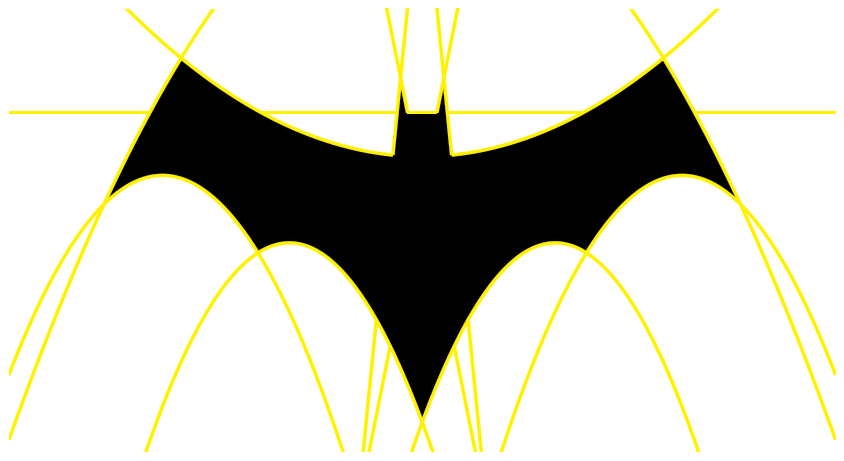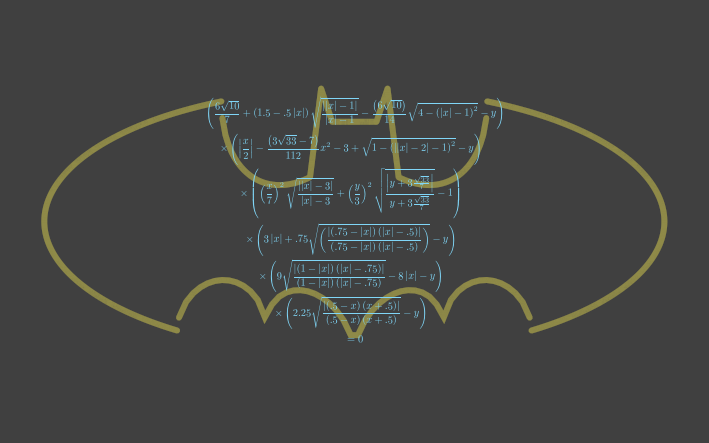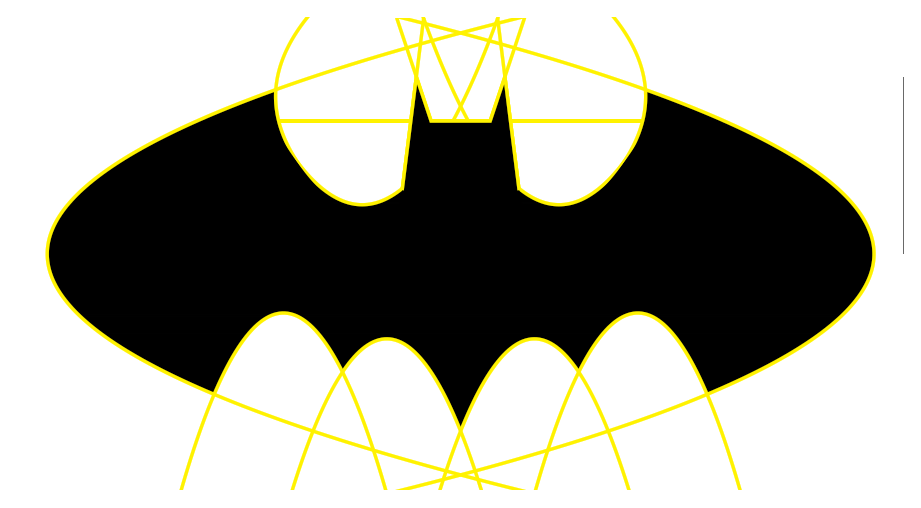loopspace

19th July 2017

# 1 Introduction

This is an end-of-term activity that I designed for some students that had recently been working on quadratics. It could be used elsewhere in the topic as its main purpose is to provide practice with plotting quadratics.

• The Instructions. I would recommend copying them two to a page. The size of the axes was determined by the graph paper that I had to hand, the extremes of the picture are at $±11$ on the $x$–axis and $-8$ to $5$ on the $y$–axis.

• The Result (simple version), The Result (elaborate version). Note that when following the instructions then the picture is (deliberately) produced upside-down.

# 2 End of Term Activities

Producing end of term activities is something that I find tricky. The echoing cry of "Can we have a fun lesson?" causes me nightmares. It's not that I can't find things that the students will think are fun, but the difficulty that I have is that so many of the resources I find have zero mathematical content.

Quite frankly, they are about to have a period of several weeks with, in all likelihood, zero mathematical content. I'd rather that their last official hour or half-hour of mathematics was not like that.

The difficulty is that the vast majority of the joy in mathematics is like that of climbing a mountain: the journey matters. Driving to the top in a car does not give the same experience as walking up the track.

So an ideal end of term activity is one that the students regard as enjoyable, but that nevertheless does involve them doing some maths. The best place to look for the intersection of those is either in recreational maths, or in practising techniques where the students already know what to do but a little extra practice wouldn't go amiss.

# 3 Designing an Activity

The inspiration for drawing something with quadratics came from a whole host of sources. The initial spark was from thinking about the ubiquitous "curves of pursuit". This activity ticks all the wrong boxes. The worst thing about it is that it is highly mathematical, but the maths is almost never exposed to the students1.

1There are a variety of mathematical ideas buried in it, probably the best is as step-by-step solutions of ordinary differential equations.

A related activity is that of curve stitching. Again, the maths is almost never involved in this activity. Proving that the envelope of the curve is a parabola involves some heavy geometry or calculus. However, there is another option with this. The way that a curve is formed by curve stitching is similar to how a quadratic Bézier curve is built from lines2.

2It might be exactly the same, I haven't looked closely enough at the constructions to compare them as yet.

Now Bézier quadratics is an interesting place to be. Béziers are important in vector graphics, meaning that a lot of drawings are done using Bézier curves to approximate more complicated curves. Most implementations (e.g., SVG, PDF, and most fonts) go up to cubic Béziers but you can get quite far with just quadratics3. Indeed, the difference between using cubic and quadratic Béziers is all about how many curves one has to use to make a decent looking drawing.

3Incidentally, a while ago there was a bit of a fuss made about the apparent fact that Twitter's logo was formed out of circles, and only two sizes of circle at that. It isn't. The official logo is available as an SVG from their branding page. The SVG specification includes the ability to draw arcs so if Twitter's logo were formed entirely from circles then every drawing command should be an arc, and those arcs should have one of two radii. Not only are no two radii exactly the same (they form three loose-knit groups), three of the curves are actually cubic Béziers, the most significant being the belly. Interestingly, the one that visually looks the least like an arc to me is the top of the upper wing which actually is an arc. Appearances can be deceptive.

So there's potential for an activity involving using quadratic Béziers to approximate a drawing and then using curve stitching to actually make the picture. But that activity involves introducing several new concepts and therefore is more suited to an exploratory afternoon rather than an end of term activity.

# 4 Designing a Picture

Nevertheless, the idea of using curves to make a picture is an attractive one. To make it an end of term activity, however, the curves need to be ones that the students already know about. So for the quadratics, it would have to be ones of the form $y=a{x}^{2}+bx+c$. Again, that isn't much of a restriction since any curve can be approximated by a bunch of those. To make it an enjoyable activity, there mustn't be too many curves and ideally the fact that they are parabolæ should be used. That means a shape with lots of arches. Bridges would be a possibility, but the Batman logo is a far more attractive proposition.

There are quite a few variations of the Batman logo which gives a lot of scope for finding one that can be drawn with parabloæ. To make the drawing, I loaded the image into the awesome Geogebra program and then fit quadratics to it. It was easiest to use the $a\left(x-p{\right)}^{2}+q$ form to fit the curves and then convert them to the $a{x}^{2}+bx+c$ form afterwards. I decided that the "head" part would be best with straight lines.

To delay the moment when a student figured out the drawing, I then turned them upside-down.

# 5 The Instructions

Draw axes with $-14\le x\le 14$ and $-9\le y\le 9$ using $1$cm per unit. Plot the following quadratics all on the same axes.

1. $y=-0.05{x}^{2}-4$

2. $y=0.1{x}^{2}-14$

3. $y=0.25{x}^{2}-4.4x+16$

4. $y=0.25{x}^{2}+4.4x+16$

5. $y=0.3{x}^{2}-2.7x+5$

6. $y=0.3{x}^{2}+2.7x+5$

Now colour in the region in the middle.

To complete the shape, plot the following lines and colour in the obvious regions.

1. $y=-10x-14$

2. $y=10x-14$

3. $y=-5x-3$

4. $y=5x-3$

5. $y=-5.5$

# 6 The Result# 7 The Batman Curve

It's impossible to post something about drawing a Batman logo without mentioning The Batman curve.There are many explanations of this equation online so as this isn't the main point of this post, I'll not give a detailed explanation here. There are some themes that would not be out of place in a classroom: factorisation appears which fits with quadratics, but dealing with the square roots is a little technical4, and absolute values are A'level.

4and really is about exploiting how computer graphics systems work rather than being about mathematics

But in principle it works in the same fashion as the quadratic logo: find some curves and lines that draw out a Batman logo and plot them. Only instead of drawing the entire curves, it only draws the parts that are necessary (this is what the square roots do), and it exploits the symmetries in the picture (this is what the absolute values do). By removing both of those artefacts, we can draw this logo by plotting a list of curves and colouring in a particular region defined by those curves.

The resulting curves are not, however, parabolæ. Several are straight lines (which is not a problem), one is an ellipse, and the others are slightly more complicated. However, using the principle that everything can be approximated by parabolæ, we can get a close approximation. The difference between this picture and the earlier one is that we have to allow parabolæ on their sides, meaning that they can be of the form $x=a{y}^{2}+by+c$.

For this logo, suitable curves are:

1. $x=.5{y}^{2}-7$

2. $x=-.5{y}^{2}+7$

3. $y=-{x}^{2}-6x-10$

4. $y=-{x}^{2}+6x-10$

5. $y=-{x}^{2}-2.5x-3$

6. $y=-{x}^{2}+2.5x-3$

7. $y=.6{x}^{2}+2x+2.5$

8. $y=.6{x}^{2}-2x+2.5$

9. $x=-.3{y}^{2}+1.6y+1$

10. $x=.3{y}^{2}-1.6y-1$

11. $y=8x+9$

12. $y=-8x+9$

13. $y=3x+.75$

14. $y=-3x+.75$

15. $y=2.25$This has considerably more curves to plot, making it less suitable for an activity to be done by hand. However, it could work as a Geogebra activity: plot the curves on Geogebra, then print it out and colour it in.